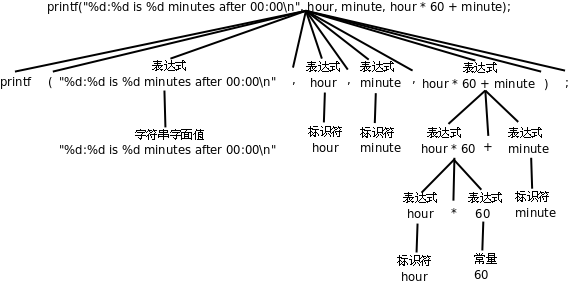## 5. 表达式

`hour * 60 + minute;`

```int total_minute;
total_minute = hour * 60 + minute;```

```int total_minute, total;
total = total_minute = hour * 60 + minute;```

（= Initializer的部分可以不写）

`printf("%d:%d is %d minutes after 00:00\n", hour, minute, hour * 60 + minute);``printf("%d:%d is %d minutes after 00:00\n", hour, minute, total_minute = hour * 60 + minute);`

`minute + 1 = hour;`

```hour = 11;
minute = 59;
printf("%d and %d hours\n", hour, minute / 60);```

⌊59/60⌋=0
⌈59/60⌉=1
⌊-59/60⌋=-1
⌈-59/60⌉=0

```printf("%d hours and %d percent of an hour\n", hour, minute * 100 / 60);
printf("%d and %f hours\n", hour, minute / 60.0);```

### 习题

1、假设变量`x``n`是两个正整数，我们知道`x/n`这个表达式的结果要取Floor，例如`x`是17，`n`是4，则结果是4。如果希望结果取Ceiling应该怎么写表达式呢？例如`x`是17，`n`是4，则结果是5；`x`是16，`n`是4，则结果是4。